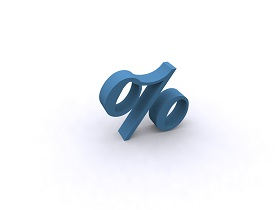## APY Calculator - Calcunation.com

Calculate the Annual Percentage Yield and how it compares with APR with this APY Calculator.

Periods per Year:
(Annual=1, Monthly=12, Weekly=52, Daily=365)

Interest Rate Per Period: %